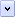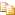Microsoft JScript functions perform actions; they can also return values. Sometimes these are the results of calculations or comparisons. Functions are also called "global methods".

Functions combine several operations under one name. This lets you streamline your code. You can write out a set of statements, name it, and then execute the entire set by calling it and passing to it any information it needs.

You pass information to a function by enclosing the information in parentheses after the name of the function. Pieces of information that are passed to a function are called arguments or parameters. Some functions do not take any arguments at all while others take one or more arguments. In some functions, the number of arguments depends on how you are using the function.

JScript supports two kinds of functions: those that are built into the language, and those you create yourself.

# Special Built-in Functions

The JScript language includes several built-in functions. Some let you handle expressions and special characters, while others convert strings to numeric values. A useful built-in function is eval(). This function evaluates any valid JScript code that is presented in string form. The eval() function takes one argument, the code to be evaluated. Here is an example using this function.Copy Code
```var anExpression = "6 * 9 % 7";
var total = eval(anExpression); // Assigns the value 5 to the variable total.
var yetAnotherExpression = "6 * (9 % 7)";
total = eval(yetAnotherExpression) // Assigns the value 12 to the variable total.
// Assign a string to totality (note the nested quotes)
var totality = eval("'...surrounded by acres of clams.'");```

You can create your own functions and use them where needed. A function definition consists of a function statement and a block of JScript statements.

The checkTriplet function in the following example takes the lengths of the sides of a triangle as its arguments. It calculates from them whether the triangle is a right triangle by checking whether the three numbers constitute a Pythagorean triplet (the square of the length of the hypotenuse of a right triangle is equal to the sum of the squares of the lengths of the other two sides). The checkTriplet function calls one of two other functions to make the actual test.

Notice the use of a very small number ("epsilon") as a testing variable in the floating-point version of the test. Because of uncertainties and round-off errors in floating-point calculations, it is not practical to make a direct test of whether the three numbers constitute a Pythagorean triplet unless all three values in question are known to be integers. Because a direct test is more accurate, the code in this example determines whether it is appropriate and, if it is, uses it.Copy Code
```var epsilon = 0.00000000001; // Some very small number to test against.

// The test function for integers.
function integerCheck(a, b, c)
{
// The test itself.
if ( (a*a) == ((b*b) + (c*c)) )
return true;

return false;
} // End of the integer checking function.

// The test function for floating-point numbers.
function floatCheck(a, b, c)
{
// Make the test number.
var delta = ((a*a) - ((b*b) + (c*c)))

// The test requires the absolute value
delta = Math.abs(delta);

// If the difference is less than epsilon, then it's pretty close.
if (delta < epsilon)
return true;

return false;
} // End of the floating-poing check function.

// The triplet checker.
function checkTriplet(a, b, c)
{
// Create a temporary variable for swapping values
var d = 0;

// First, move the longest side to position "a".

// Swap a and b if necessary
if (b > a)
{
d = a;
a = b;
b = d;
}

// Swap a and c if necessary
if (c > a)
{
d = a;
a = c;
c = d;
}

// Test all 3 values. Are they integers?
if (((a % 1) == 0) && ((b % 1) == 0) && ((c % 1) == 0))
{
// If so, use the precise check.
return integerCheck(a, b, c);
}
else
{
// If not, get as close as is reasonably possible.
return floatCheck(a, b, c);
}
} // End of the triplet check function.

// The next three statements assign sample values for testing purposes.
var sideA = 5;
var sideB = 5;
var sideC = Math.sqrt(50.001);

// Call the function. After the call, 'result' contains the result.
var result = checkTriplet(sideA, sideB, sideC);```

Home